Next: Generating and from and Up: The secondary Trust-Region subproblem Previous: The secondary Trust-Region subproblem   Contents

# Generating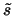.

Let us define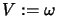,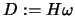,, equation 5.8, can now be rewritten: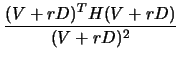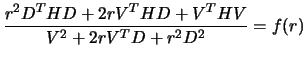We will now search for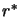, root of the Equation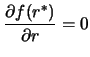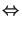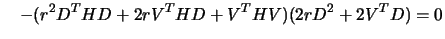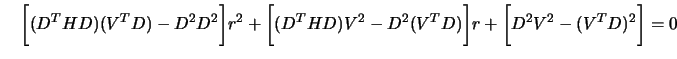(Using the fact that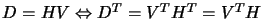.)
We thus obtain a simple equation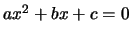. We find the two roots of this equation and choose the onewhich maximize 5.8.is thus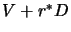.

Frank Vanden Berghen 2004-04-19